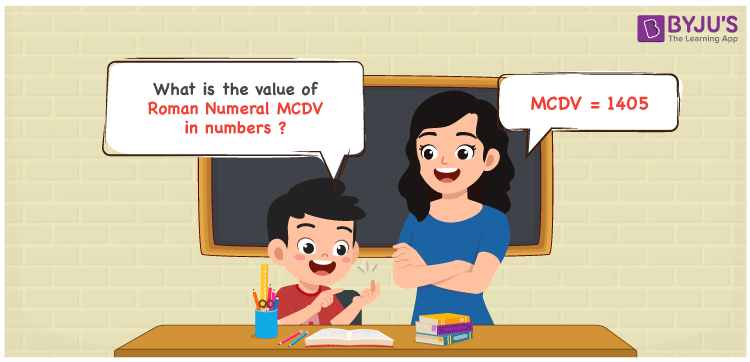Checkout JEE MAINS 2022 Question Paper Analysis : Checkout JEE MAINS 2022 Question Paper Analysis :

# MCDV Roman Numerals

MCDV Roman Numerals is 1405. This article gives a wide knowledge of converting the roman numerals MCDV in numbers as 1405. Roman numerals have to be learnt well in elementary education to avoid confusions in higher education levels. These roman numerals conversion materials aim to offer a strong foundation of fundamental topics among students. Therefore, MCDV can be indicated as 1405 in numbers.

 Number Roman Numeral 1405 MCDV## How to Write MCDV Roman Numerals in Numbers?

Obtain an in-depth idea of the process used to write MCDV to numbers using the steps given here.

MCDV = M + (D – C) + V

MCDV = 1000 + (500 – 100) + 5

MCDV = 1405

## Video Lesson on Roman Numerals## Frequently Asked Questions on MCDV Roman Numerals

### Why should 1405 be written as MCDV?

In roman numerals

1000 is written as M

400 is written as CD

5 is written as V

1405 = M + CD + V = MCDV

### Find 1000 + 405.

We know that

1000 + 405 = 1405

Hence, 1000 + 405 is 1405 which is written as MCDV.

### Find the remainder we get when MCDV is divided by IX.

We know that

MCDV = 1405

IX = 9

So the remainder we get when MCDV is divided by IX is I.